# Still more new Ducks and Kaliset variations

Kali found another new formula for the kaliset in this Fractalforums post.

z=abs(z*c+1)+1/abs(z*c+1)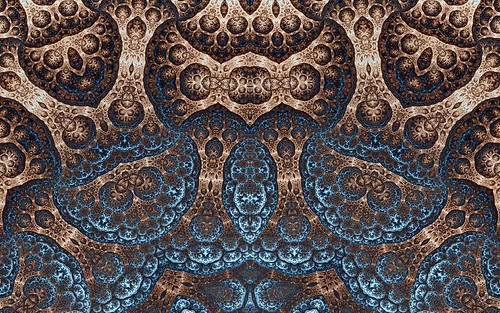z=(z*c+1)+1/(z*c+1)I played around with adding a complex log call to the formulas and this also gives unique results. The log call brings the space filling shapes back into the original Ducks Fractal territory.

z=abs(log(z*c+1))+1/abs(log(z*c+1))z=log(abs(z*c+1))+1/log(abs(z*c+1))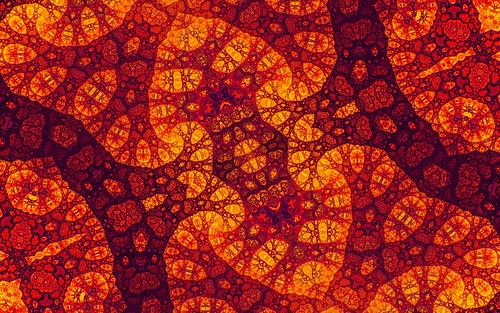These new formulas are now included in Visions Of Chaos.

Jason.

# More Ducks and Kaliset variations

Following on from the last post about variations to the Ducks and Kaliset fractals, here are a few more.

z=1/abs(z)+cz=abs(z)/abs(c)+cz=abs(z)^-3+c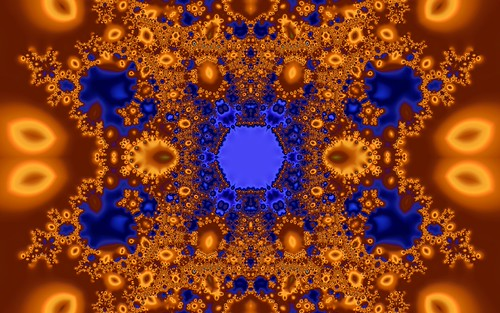z=abs(z^-3)+c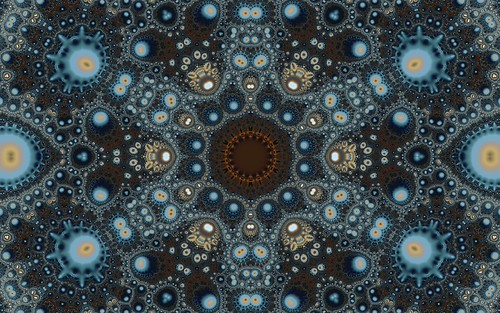z=abs(z)/c+c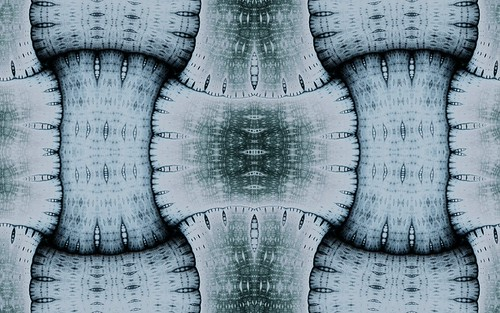z=abs(z/c)+cAfter some experiments with implenting orbit traps on Ducks and Kaliset fractals, the cross orbit trap seems to give the best results so far.There are much higher resolution images of the above in my Ducks Fractals Flickr Gallery.

All of these variations are now included with Visions Of Chaos.

Jason.

# Kalisets and Hybrid Ducks

I saw a new Ducks fractal variation named Kalisets in this fractalforums post by Kali.

The new variation uses the formula z=abs(z^-1)+c. It turns out this is actually the burning ship formula with power -1 rather than power -2. Like the Ducks fractals the “Mandelbrot” variety (c is set to the value of each pixel prior to iterating) of this formula gives rather boring images, but the “Julia” variety (c is constant across the image) leads to new interesting results.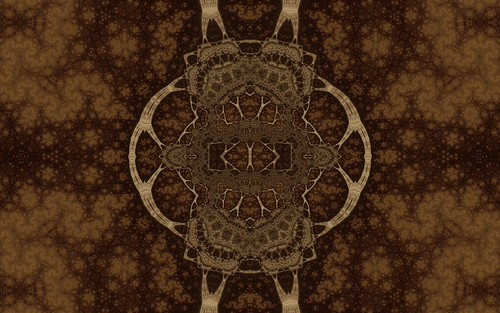After trying different variaitons of the formula I came up with these three.

z=log(abs(z^-1)+c)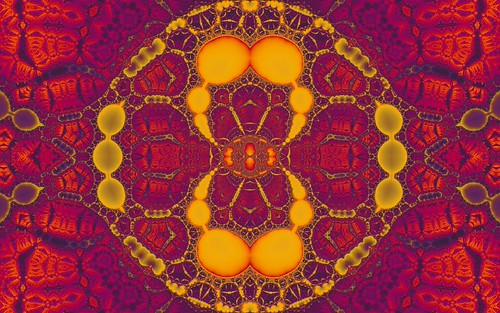z=log(sin(abs(z^-1))+c)z=sin(abs(z^-1))+c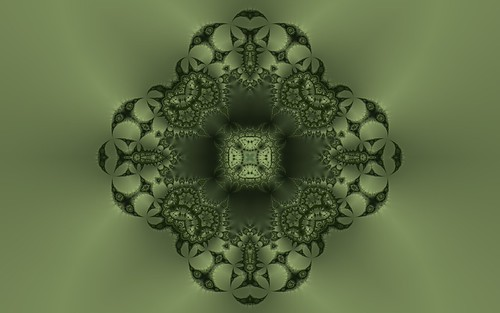Using the hybrid fractal principal also works fine with these formulas. For the hybrid fractals the formula, variation and power are alternated between iterations.Higher resolution images are avilable in my flickr Ducks set.

The most interesting thing about these new types of fractals is how the Mandelbrot versions are boring but the Julia versions give rise to these complex patterns and structures. People must have tried these formulas in the past but possibly once they saw the boring Mandelbrot version they gave up and tried other formulas. There are bound to be many more formulas based on these principals found in the future.

The above new variations are now included in the latest version of Visions Of Chaos.

Jason.